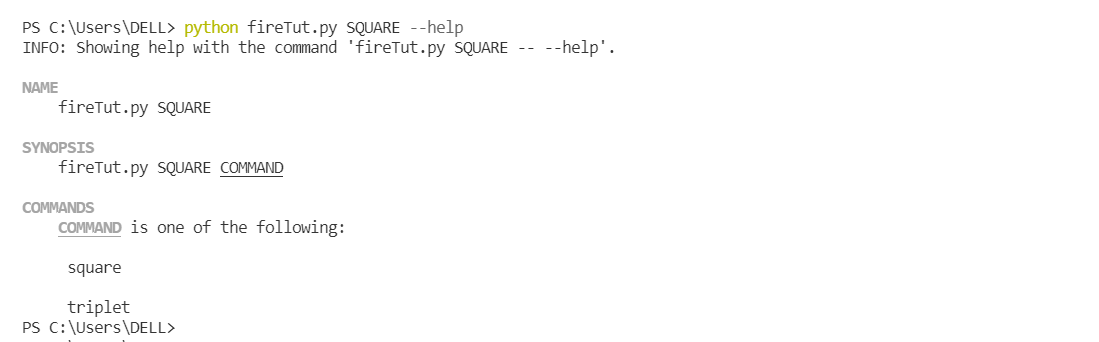# Python Fire Module

• Last Updated : 28 Nov, 2021

Python Fire is a library to create CLI applications. It can automatically generate command line Interfaces from any object in python. It is not limited to this, it is a good tool for debugging and development purposes. With the help of Fire, you can turn existing code into CLI. In this article, we will see the use of fire.

## Installation

Firstly, there are different installing methods that you can perform according to your choice.

Install using pip:

`pip install fire`

The Fire works on any Python object including components, dictionaries, lists, tuples, etc.

## Calling Fire on Function

Here’s an example of calling fire on a function for this we will make a function and call the function in CLI.

## Python3

 `import` `fire ` ` `  `def` `greeting(name): ` `    ``return` `"Hello "` `+` `name ` ` `  `fire.Fire(greeting(``"World!"``)) `

Output:

`Hello World!`

Then, from the terminal, you can run using the following commands:

` python main.py`

## Setting a Function as Entry Point

There is another way to run this program by setting a function in the Fire function at invoking time. By doing so, we only need to pass arguments at runtime without telling which method to call.

## Python3

 `import` `fire ` ` `  `def` `hello(name): ` `    ``return` `"Hello "` `+` `name ` ` `  `if` `__name__ ``=``=` `'__main__'``: ` `    ``fire.Fire(hello)`

Output:

`Hello world`

If you run this in the terminal using the below command, it will give the same result as the above function. Calling Fire consumed name = “World”. Using this method of calling fire, saves from writing the function name with arguments.

`python main.py world`

Both the ways of calling will give “Hello World” as an output.

## Calling Function on Classes

Here is an example class created named Square and a method “square” is defined inside it. Once, we call fire on any class, we can access it as a method on the command line directly. We will see how to call this method using the below example,

## Python3

 `import` `fire ` ` `  `class` `Square(``object``): ` ` `  `    ``def` `square(``self``, number): ` `        ``return` `number``*``number ` ` `  `fire.Fire(Square)`

Output:

`4`

There are two ways to execute this class, the first method is:

`python main.py square 2`

The second way which will give the same desired output is:

`python main.py square --number=2`

Both the methods will give the same result = 4.

## Passing Arguments to Command

Fire is convenient as it allows to pass arguments from a command line. In the above example, we invoked the method from the command line and assigned arguments at the same time.

### Using Hierarchical commands

Fire can be used to instantiate classes inside the class, creating a hierarchical view. let’s take an example,

## Python3

 `import` `fire ` ` `  `class` `Calculator(``object``): ` `    ``def` `__init__(``self``): ` `        ``self``.SQUARE ``=` `Square() ` ` `  `class` `Square(``object``): ` ` `  `    ``def` `square(``self``, number): ` `        ``return` `number``*``number ` `       `  `    ``def` `triplet(``self``,number): ` `        ``return` `number``*``number``*``number ` ` `  `fire.Fire(Calculator) `

In this way, the Calculator class instantiates the square class and its object is created. The member functions of the instantiated class can be accessed at run time like:## Fire Flags

To know more about fire commands associated with a particular object or class use –help flag with the object or class. For Example, shown below, we are querying about the “SQUARE” object, and it shows two commands associated with it – “square” and “triplet”. This means you can only pass these two commands with SQUARE with their parameters.## Why Fire?

• Fire makes code more readable.
• Easy to create command-line Interfaces.
• fire.Fire() executes the full content of the program when invoked at the end of a program.

My Personal Notes arrow_drop_up
Recommended Articles
Page :